Next: How to pick inside Up: The Trust-Region subproblem Previous: Finding the root of   Contents

# Starting and safe-guarding Newton's method

In step 1 of Newton's method, we need to find a value of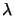such thatand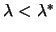. What happens if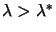(or equivalently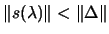)? The Cholesky factorization succeeds and so we can apply 4.21. We get a new value forbut we must be careful because this new value can be in the forbidden region.
If we are in the hard case, it's never possible to get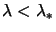(or equivalently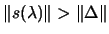), therefore we will never reach point 2 of the Newton's method.
In the two cases described in the two previous paragraphs, the Newton's algorithm fails. We will now describe a modified Newton's algorithm which prevents these failures:
1. Compute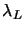and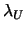respectively a lower and upper bound on the lowest eigenvalue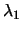of.
2. Choose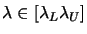. We will choose:3. Try to factorize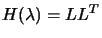(if not already done).
• Success:
1. solve2. Compute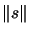:
•: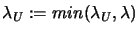We must be careful: the next value ofcan be in the forbiddenregion. We may also have interior convergence: Check:
•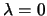: The algorithm is finished. We have found the solution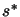(which is inside the trust region).
•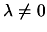: We are maybe in the hard case. Use the methods described in the paragraph containing the Equation 4.15 to find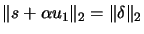. Check for termination for the hard case.
•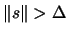: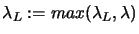3. Check for termination for the normal case:is on the boundary of the trust region.
4. Solve5. Compute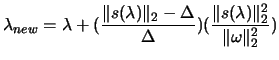6. Check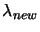: Try to factorize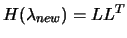• Success: replaceby• Failure: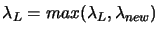The Newton's method, just failed to choose a correct. Use the alternative'' algorithm: pickinside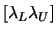(see Section 4.5 ).
• Failure: Improveusing Rayleigh's quotient trick (see Section 4.8 ). Use the alternative'' algorithm: pickinside(see section 4.5 ).